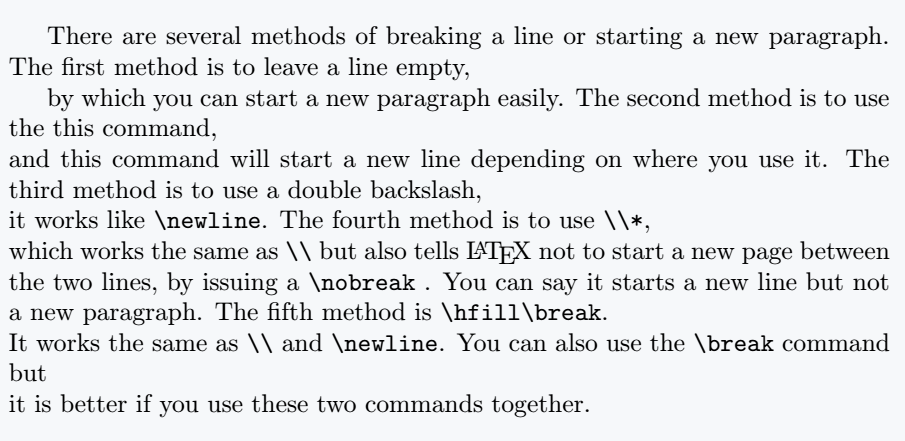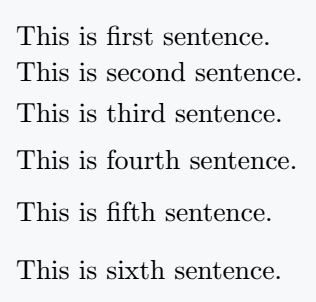# How do you make a line break or new line in LaTeX?

New line, new paragraph start or line break in a document are usually required by us. There are several ways to do this in Latex. In this tutorial, we will discuss all methods of starting a new line, new paragraph, or line break in LaTeX.

## Line break in LaTeX

There are several methods of breaking a line or starting a new paragraph.

1. first method is to leave a line empty, by which you can start a new paragraph easily.

2. second method is to use the \newline command, this command will start a new line depending on where you use it.

3. third method is to use a double backslash \\, it works like \newline.

4.  fourth method is to use \\* , which works the same as \\ but also tells LaTeX not to start a new page between the two lines, by issuing a \nobreak . You can say it starts a new line but not a new paragraph.

5. fifth method is to combine two commands \hfill\break. It works the same as \\ and \newline. You can also use the \break command but it is better if you use these two commands together.

\documentclass{article}
\begin{document}
There are several methods of breaking a line or starting a new paragraph.
The first method is to leave a line empty,

by which you can start a new paragraph easily.
The second method is to use the this command,\newline
and this command will start a new line depending on where you use it.
The third method is to use a double backslash, \\
it works like \verb|\newline|. The fourth method is to use \verb|\\*|,  \\*
which works the same as \verb|\\| but also tells \LaTeX\;not to start a new page between the two lines, by issuing a \verb|\nobreak| . You can say it starts a new line but not a new paragraph.
The fifth method is \verb|\hfill\break|.\hfill\break
It works the same as \verb|\\| and \verb|\newline|. You can also use the \verb|\break| command but \break
it is better if you use these two commands together.
\end{document}

Output :Another method of line breaking is to use the \linebreak[] command. But the specialty of this command is that you can pass arguments to the command to represent the property, which is 0 to 4.

If you use \linebreak with no arguments, the command will take 4 as the default argument. Here argument 0 means line break can be easily ignored and 4 means it must be done anyway. And when the line break option is used LaTeX will try to give you the best result.

\documentclass{article}
\begin{document}
This is first sentence.
This is second sentence.
This is third sentence.\linebreak
This is fourth sentence.
This is fifth sentence.
This is sixth sentence.
\end{document}

Output :But when  is passed as an argument to this command the output will be like this. Meaning line break will be ignored.## Vertical space between two lines

With the above methods, you can only break lines but cannot specify the vertical space between two lines. But if you want to manually specify vertical space for next line after line break then, you need to use \\[<len>] or \\*[<len>] . And in this [<len>] you can pass length as an argument for how much vertical space you need.

\documentclass{article}
\begin{document}
This is first sentence.\\[1pt]
This is second sentence.\\[3pt]
This is third sentence.\\[5pt]
This is fourth sentence.\\[7pt]
This is fifth sentence.\\*[9pt]
This is sixth sentence.\\*[11pt]
\end{document}

Output :